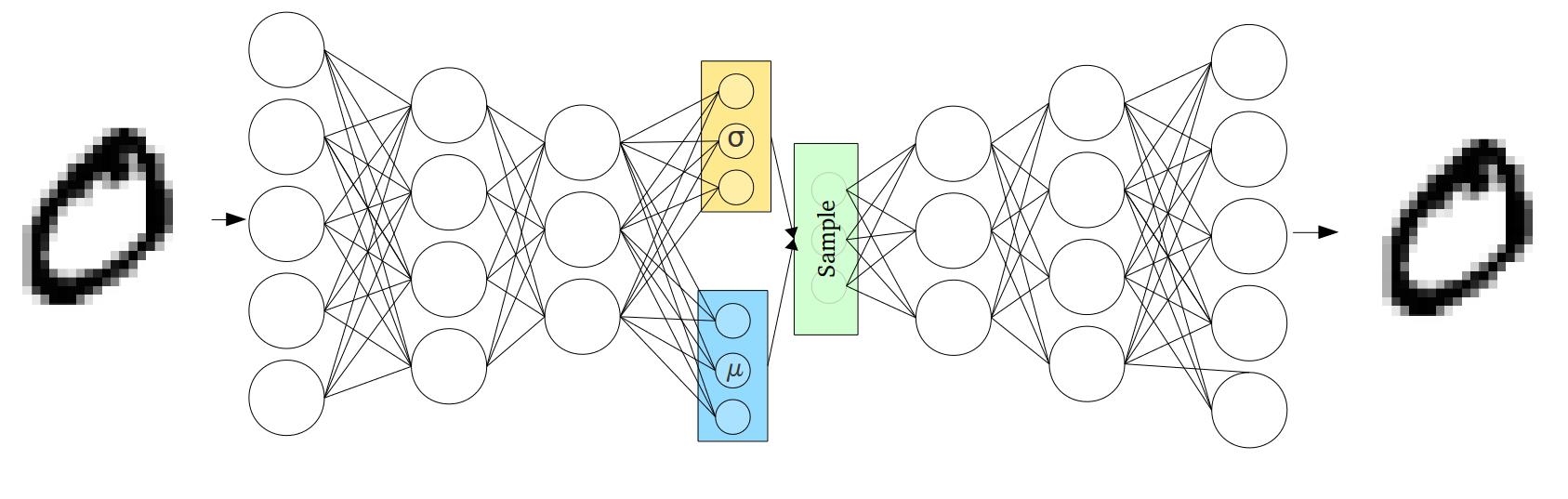April 7th, 2019

### Days 82 - 91: Generative ModelsBy Aidin

In this blog, we study the generative models and why do we need these models. Also, we will summarize different generative models and discuss their differences and similarities.

In statistics and machine learning, two main areas are called the generative approach and the discriminative approach.

Let 𝑿 be the observable variable and 𝒚 be the target variable, then:

• A generative model is a statistical model of the joint probability distribution on 𝑿×𝒚, 𝑷(𝑿,𝒚),
• A discriminative model is a model of the conditional probability of the target 𝒚, given an observation 𝑿,  𝑷(𝒚|𝑿=𝒙).

In classification tasks, one can use a discriminative model 𝑷(𝒚|𝑿=𝒙) directly or use a generative model and compute the conditional probability from that.

Another application of the generative models is to find the conditional distribution of the 𝑿 given 𝒚. This means a generative model can be used to "generate" random instances.

The examples of generative models are:

• Mixture models (e.g. Gaussian Mixture Model)
• Hidden Markov Model
• Bayesian Network Model
• Restricted Boltzmann Machine
• Variational Autoencoder

Mixture Models:In simple words, a mixture model is a weighted sum of distributions. In this model, we try to fit K distributions to a data set. Therefore, apart from knowing the best value for K, we have to find the parameters of every distribution as well as the weight of every distribution in the summation. This procedure is usually done by an expectation maximization method in which every iteration consists of two steps where at one step the parameters are fixed and the weights are optimized while on the other step it is vice versa.

https://stephens999.github.io/fiveMinuteStats/intro_to_mixture_models.html

Hidden Markov Model:Hidden Markov Model (HMM) is a statistical Markov model in which the system being modeled is assumed to be a Markov process with unobserved (i.e. hidden) states. This video explains the HMMs very well:

HMMs are widely used for handwriting detection.

Bayesian Network Model:

A Bayesian network is a probabilistic graphical model that represents a set of variables and their conditional dependencies via a directed acyclic graph. The following is a nice example of a Bayesian network:A simple Bayesian network. Rain influences whether the sprinkler is activated, and both rain and the sprinkler influence whether the grass is wet. Using this graph we can find the joint distribution of sprinkler, rain, and wet grass.

Restricted Boltzmann Machine:

This is a neural network model that finds the interdependencies between the input features and hidden nodes of the neural network. The following is a good representation of this model:One of the applications of this method is in the recommendation systems.

Variational Autoencoder:

When using generative models, you could simply want to generate a random, new output, that looks similar to the training data, and you can certainly do that too with VAEs. But more often, you’d like to alter, or explore variations on data you already have, and not just in a random way either, but in a desired, specific direction. This is where VAEs work better than any other method currently available.

A VAE architecture looks as follows:Check this video for a good explanation:

A generative adversarial network is a class of machine learning systems. Two neural networks contest with each other in a zero-sum game framework. This technique can generate photographs that look at least superficially authentic to human observers, having many realistic characteristics.

This is how a GAN look like in general:Recent papers in GAN generate such fake images that are very difficult to detect whether they are real or fake:In my next blogs, I will focus more on GANs.